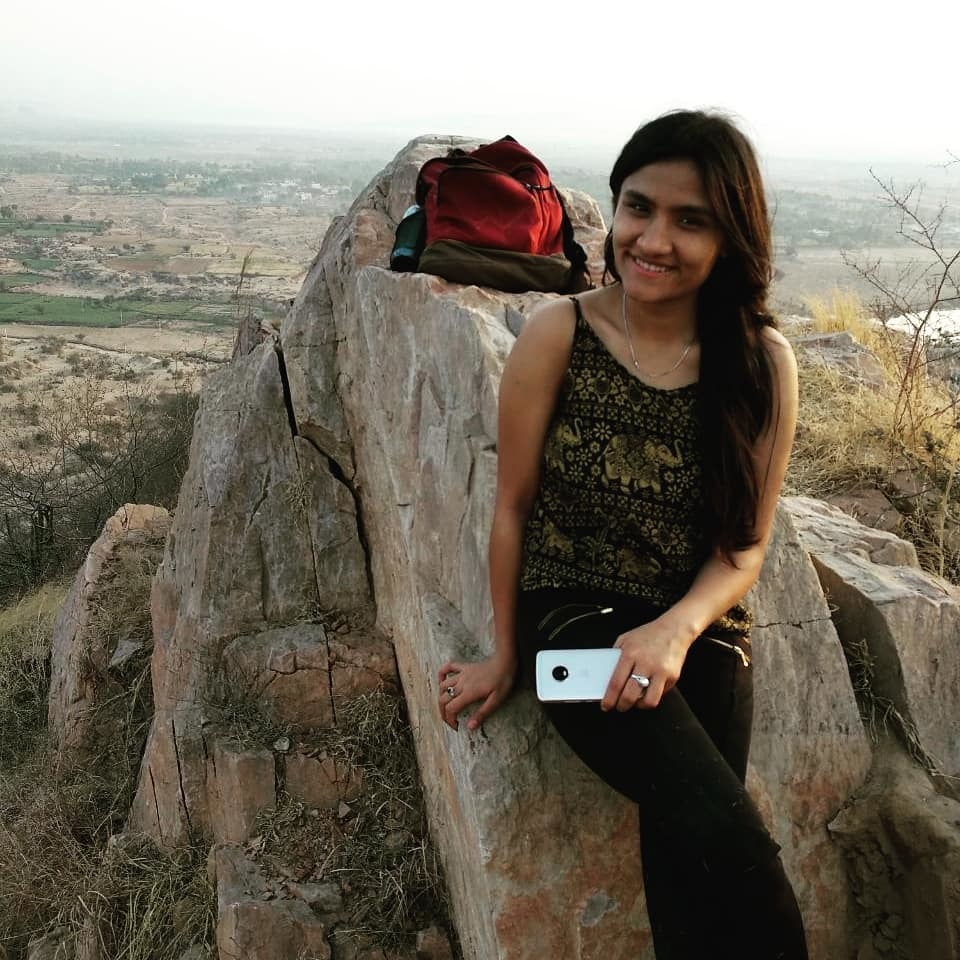# Microsoft Interview SDE intern 2019

Round 1:

There were 3 questions in the online round .2 questions related to simple array manupulation and one bst.

Those who did atleast 2 questions were selected for  other rounds.

Round 2:

It was group fly round where in we were given 2 questions.

1st : There is an array which is sorted but rotated at one end. you have to find the given element in that array.

Expected time complexity : logn.

I gave the solution using binary search.

2nd question :

Check given tree is BST .

Round 3:

Technical round :

He asked me about my project which i have done till now.I just explained him about my python web scrapping project.Then he went for questions.

1st : you are given a bst, you have to print the sum of all nodes whose value is greater than or equal to the present node.(this has to be done for every node present in the bst)

He asked me to write the code on paper.

my approach : First calculate the total sum of nodes in the bst using inorder recursive function.

initialize prev_sum=0;

Again traverse the tree in inorder, maintain a sum_prev which is adding node values.Print sum-prev_sum.   prev_sum+=node_value

int SUM=0;

void sum(Node* root)

{

if(root==NULL)

return;

sum(root-left)

SUM+=root->data;

sum(root->right)

}

int prev_sum=0;

void solution(Node* )

{

if(root==NULL)

return;

solution(root->left)

print(sum-prev_sum)

prev_sum+=root->data;

solution(root->right);

}

he asked me to try it in one loop.I gave it in one loop using : https://www.geeksforgeeks.org/add-greater-values-every-node-given-bst/

2nd question based on kadane’s algorithm.https://www.geeksforgeeks.org/largest-sum-contiguous-subarray/

Round 4:

1st question minimum length of maximum sum array.

I gave this solution using kadane’s algorithm with maintaining prev_minimum_length as a variable

2nd question : right view of binary tree

i gave my solution using level order (queue)

https://www.geeksforgeeks.org/print-right-view-binary-tree-2/

She asked me to write the code on paper.

Round5:

Senior SDE Engineer took my interview.

He first asked about me and some random stuffs, hobies and all.

then he asked me one question :

Given n points in a globe/2 dimensional infinite matrix . Find the closest point to a given point.

o(n2) solution not expected.

I approached it by making some tiles for every point . Then i told him that we can first search it in near 8 sqaures /tiles near that given point.Then he took out some mistake and asked me to think more as i m going in the right direction.

Then I told him that i ll sort the points according to x variable and then y and then restrict my search for the closest point in the region bounded by that points .

he told me not to code as i have coded in all the rounds.

My all interviews went quite well and results came after 15 days and I was selected.

Just focus on interview specific questions, Operating systems, DBMS, and data structures .

Before going for interviews Read some interview experiences .

Thanks you  geeksforgeeks for such a good platform to prepare for interviews.

Write your Interview Experience or mail it to contribute@geeksforgeeks.org

My Personal Notes arrow_drop_upCheck out this Author's contributed articles.

If you like GeeksforGeeks and would like to contribute, you can also write an article using contribute.geeksforgeeks.org or mail your article to contribute@geeksforgeeks.org. See your article appearing on the GeeksforGeeks main page and help other Geeks.

Please Improve this article if you find anything incorrect by clicking on the "Improve Article" button below.